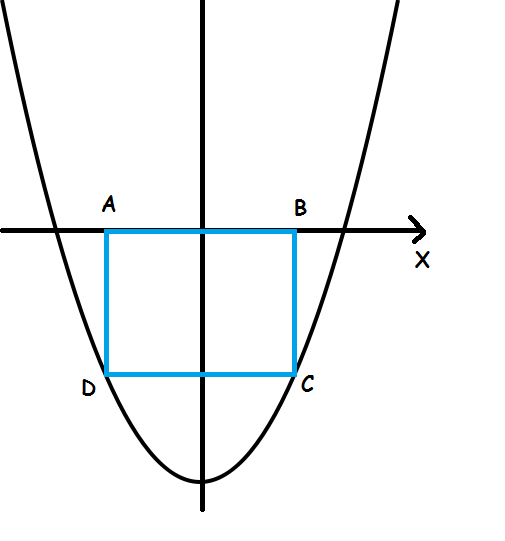# The easiest IIT JEE Problem.

Geometry Level 4If graph of the expression y = $x^{2}$ - 8x + 12 is shown in the figure then area of the square ABCD inscribed between parabola and X axis is given by:

×